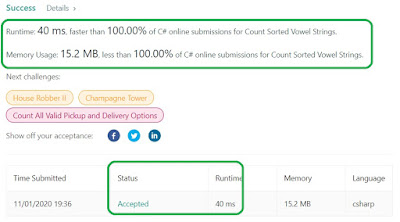## Posts

Showing posts from November, 2020

### The Holy-Grail Formula For Primes GenerationStudents at the University of Buenos Aires have published a very-short paper this month (November, 2020) providing an astonishing formula to generate all primes - in sequence. This has been the Holy Grail in Number Theory: is there a way to generate all the prime numbers one by one with a deterministic formula? The students have proved that there is. Their paper is here:  2010.15882.pdf (arxiv.org) The formula is actually very simple. Here it is: And F1 should be a constant which "magically" is F1 = 2.9200509773161350318170795639 This constant is being called now the "Buenos Aires Prime Constant". The only problem is how to find this constant without knowing a priori all the primes. This is the current open problem. If someone can extend this constant to a very-very-very large number of decimal points, the problem of primes generation will be solved. With the current one, though, it is good enough to generate few primes, in order. The code below (using Miller-Rabin

### Changing the root of a binary treeIf you work with graphics and eventually you want to pivot a binary tree using a different node as the root, this might be interesting to you. Here is the problem:  Change the Root of a Binary Tree - LeetCode 1666. Change the Root of a Binary Tree Medium 6 14 Add to List Share Given the  root  of a binary tree and a  leaf  node, reroot the tree so that the  leaf  is the new root. You can reroot the tree with the following steps for each node  cur  on the path  starting from the  leaf  up to the  root ​​​  excluding the root : If  cur  has a left child, then that child becomes  cur 's right child. Note that it is guaranteed that  cur  will have at most one child. cur 's original parent becomes  cur 's left child. Return  the new root  of the rerooted tree. Note:  Ensure that your solution sets the  Node.parent  pointers correctly after rerooting or you will receive "Wrong Answer".   Example 1: Input: root = [3,5,1,6,2,0,8,null,null,7,4], leaf = 7 Output: [7,2,nul

### Minimum Operations to Reduce X to ZeroProblem is here:  Minimum Operations to Reduce X to Zero - LeetCode From the get-go, the main technique to be used here will be a BFS (Breadth-First-Search). No need to use weights, Dijkstra. Simple BFS should do it. The one, and very important point to bare in mind: when the bug lands on a position P, it does not mean that the bug can never land on P again. Remember that the bug might have come from a forward move, or might have come from a backwards move, and these are distinct, hence when you determine the key to mark P as visited, you actually have two distinct keys: P + ForwardMove P + BackwardsMove Doing so is the key to solving it. Code is down below, cheers, ACC. public class QueueItem { public int position; public bool forwardMove; public int steps; public int key; public QueueItem(int position, bool forwardMove, int steps) { this.position = position; this.forwardMove = forwardMove; this.steps = steps; this.key = positio

### Post Number 400: BFS + DFSThis is my post number #400. I think I have this idea that when I retire I'm going to read all this shit... Illusion...  In any case, here is the problem:  Correct a Binary Tree - LeetCode 1660. Correct a Binary Tree Medium 13 2 Add to List Share You have a binary tree with a small defect. There is  exactly one  invalid node where its right child incorrectly points to another node at the  same depth  but to the  invalid node's right . Given the root of the binary tree with this defect,  root , return  the root of the binary tree after  removing  this invalid node  and every node underneath it  (minus the node it incorrectly points to). Custom testing: The test input is read as 3 lines: TreeNode root int fromNode  ( not available to  correctBinaryTree ) int toNode  ( not available to  correctBinaryTree ) After the binary tree rooted at  root  is parsed, the  TreeNode  with value of  fromNode  will have its right child pointer pointing to the  TreeNode  with a value of  toNode .

### Sometimes it is easier to solve the inverse of the problem